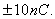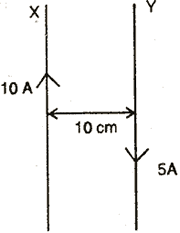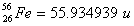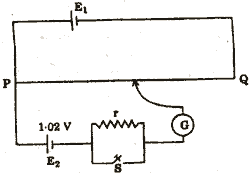Wednesday 12th August 2020

CBSE Guess > Papers > Question Papers > Class XII > 2004 > Physics > Outside Delhi Set-III

PHYSICS (Set III—Outside Delhi)

Except for the following questions, all the remaining questions have been asked in Set I and Set II.

Q. 2. Why is ground wave transmission of signals restricted to a frequency of 1500 kHz? 1

Q. 4. Draw the voltage-current characteristic of a p-n junction diode in forward bias. 1

Q. 6. Draw a ray diagram of an astronomical telescope in the normal adjustment position. What will be the length of the telescope for this position? 2

Q. 8. An electric dipole of length 4 cm, when placed with its axis making an angle of 300 with a uniform electric field experiences a torque of 4 Nm. Calculate the (i) magnitude of the electric field. (ii) potential energy of the dipole, If the dipole has charges of2

Q. 11. Two long parallel straight wires X and Y separated by a distance of 10cm in air carry currents of 10 A and 5 A respectively in the same direction. Calculate the magnitude and direction of the force on a 10 cm length of the wire Y. 2A circular coil of 200 truns, radius 10 cm carries a current of 5 A. It is suspended vertically in a uniform horizonatal fiel of 0.4 T. the field lines making and angle 600 with the plane of the coil. Calculate the magnitude of the troque that must be applied on it to prevent it form turing.

Q. 12. AT.V. tower has a height of 500 m at a given place. Calculate its coverage range, if the radius of the earth is 6400 km. 2

Q. 14. Calculate the binding energy per nucleon of nucleus.
[Given: mass ofmass of a neutron = 1.008665 u
mass of a proton =1.007825 u] 3

Q. 19. State Faraday’s laws of electrolysis. Deduce the relation connecting chemical equivalent, electrochemical equivalent and Faraday. 3

Q. 21. Potentiometer wire, PQ of 1 m length is connected to a standard cell E1. Another cell, E2 of e.m.f. 1.02 V is connected as shown in the circuit diagram with a resistance ‘r’ and a switch, S. With switch, S open, null position is obtained at a distance of 51 cm from P. Calculate (i) potential gradient of the potentiometer Wire and (ii) e.m.f of the cell E1. (iii) When switch S is closed, will null point move towards P or towards Q? Give reason for your answer. 3Physics 2004 Question Papers Class XII Delhi Outside Delhi Compartment Delhi Compartment Outside DelhiSet ISet ISet ISet ISet IISet IISet IISet IISet IIICBSE 2004 Question Papers Class XII